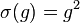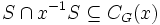# Endomorphism sends more than three-fourths of elements to squares implies abelian

This article is about a result whose hypothesis or conclusion has to do with the fraction of group elements or tuples of group elements satisfying a particular condition.The fraction involved in this case is 3/4
View other such statements

## Statement

Suppose$G$ is a finite group and$\sigma$ is an endomorphism of$G$. Suppose the subset$S$ of$G$ given by:$S := \{ g \in G \mid \sigma(g) = g^2\}$

has size more than$3/4$ of the order of$G$. Then,$G$ is an abelian group (in particular, a finite abelian group) and$\sigma$ sends every element of$g$ to its square.

## Facts used

1. The set of elements that commute with any fixed element of the group, is a subgroup: the so-called centralizer of the element.
2. The set of elements that commute with every element of the group, is a subgroup: the so-called center of the group
3. Subgroup of size more than half is whole group
4. Abelian implies universal power map is endomorphism
5. The image of a generating set under a homomorphism completely determines the homomorphism.

## Proof

This proof uses a tabular format for presentation. Provide feedback on tabular proof formats in a survey (opens in new window/tab) | Learn more about tabular proof formats|View all pages on facts with proofs in tabular format

### Proof details

Given: A finite group$G$, an endomorphism$\sigma$ of$G$.$S$ is the subset of$G$ comprising those$g$ for which$\sigma(g) = g^2$. We are given that$\! |S| > (3/4) |G|$.

To prove:$G$ is abelian and$S = G$, i.e.,$\sigma(g) = g^2$ for all$g \in G$.

Proof: We initially focus on a single element$x \in S$ and try to show that its centralizer is the whole of$G$. We then shift focus to$S$ as a set, show that it is contained in the center, and since the center is a subgroup, it is forced to be all of$G$.

Step no. Assertion/construction Facts used Given data used Previous steps used Explanation Commentary
1 Suppose$x,y \in G$ are such that$x,y,xy$ are all in$S$. Then$xy = yx$ -- Definition of$S$ as the set of elements mapped to their squares,$\sigma$ is an endomorphism [SHOW MORE] Convert local property of elements going to squares to local property of pairs commuting.
2 For any$x \in S$, define$x^{-1}S := \{ x^{-1}s : s \in S \}$. We have$S \cap x^{-1}S \subseteq C_G(x)$, where$C_G(x)$ is the centralizer of$x$ in$G$. Step (1) [SHOW MORE] Toward establishing a large centralizer for fixed but arbitrary element of$S$ -- use local property of elements going to squares.
3 For any$x \in S$, the intersection$S \cap x^{-1}S$ has size greater than$|G|/2$$|S| > (3/4)|G|$ [SHOW MORE] Toward establishing a large centralizer for fixed but arbitrary element of$S$ -- use size constraints and combinatorial formula.
4 For any$x \in S$, the subgroup$C_G(x) = \{ y \in G \mid xy = yx \}$ is equal to the whole group$G$ Facts (1), (3) Steps (2), (3) [SHOW MORE] Complete establishing that a fixed but arbitrary element of$S$ has centralizer the whole group -- use that big enough subgroups must contain everything.
5$S$ is contained in the center$Z(G)$ of$G$ Step (4) [SHOW MORE] Shift focus to$S$ as a set.
6$G$ equals its own center, hence is abelian Facts (2), (3)$|S| > (3/4)|G|$ Step (5) [SHOW MORE] Clinch -- use that big enough subgroups must contain everything.
7$S$ is a generating set for$G$. Fact (3)$|S| > (3/4)|G|$ -- [SHOW MORE]
8$S = G$, i.e.,$\sigma(g) = g^2$ for all$g \in G$. Facts (4), (5) -- Steps (6), (7) [SHOW MORE]

Steps (6) and (8) clinch the proof.